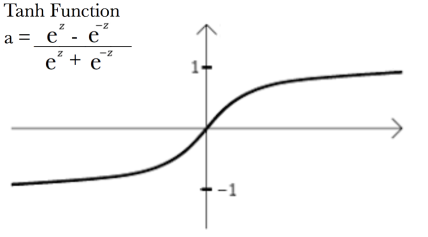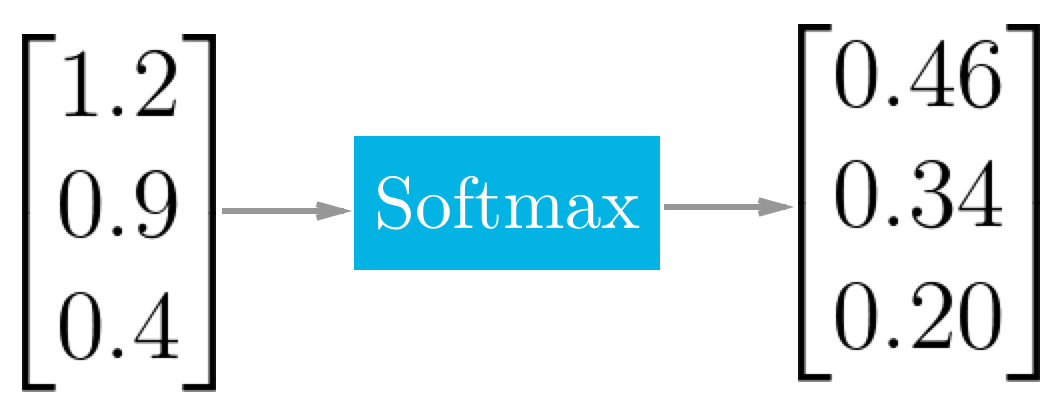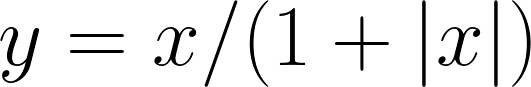# What is Deep Learning ?

Deep Learning is a subset of machine learning in artificial intelligence. It is an artificial intelligence (AI) function that imitates the workings of the human brain in processing data for decision making. Learning can be supervised ,unsupervised or semi-supervised. Deep learning is also known as Deep Learning Neural Network or Deep Neural Learning. Deep learning can work for structured as well as unstructured data. In many research areas, deep learning is used for unstructured data such as image, audio etc. but it can also used for structured data.

Deep learning is best applied to unstructured data like images, video, sound or text. An image is collection of pixels, text is collection of words or characters, video is collection of images and sound is bunch of time series data. This data is not organized in a typical relational database by rows and columns. That makes it more difficult to specify its features manually.

# Deep Learning Vs Neural Network

Neural network consist of only three layers : Input, Hidden & Ouput Layer. A neural network consists of more than three layers including input and output layers is known as Deep Learning Neural Network where the hidden layer becomes deeper. The “deep” in deep learning is referring to the depth of layers in a neural network and therefore hidden layer becomes deeper in deep learning algorithm.During training time, values of input & output layer is known to system and values of hidden layer is unknown. The target of training process is to find the unknown values of hidden layer. Mathematically input layer consists of different input values X={x1,x2,x3, ......xn} and for each input value corresponding output value is present in output layer Y={y1,y2,y3,.....yn} i.e. y1 output corresponds to input x1 , y2 output corresponds to input x2 and so on. Each input value can be a scalar value or vector or matrix but each output value is always a scalar value. System becomes complex when number of hidden layer is greater than 1. Deep learning solves this complexity by using different types of architecture.

# Types of Hidden Layer

Other than Input and Output Layer, there are different types of hidden layers that helps to perform transformation of data or features of previous layer to generate data or features for next layer.
• Convolutional Layer
• Dense or Fully connected Layer
• Dropout Layer
• Pooling Layer
• Normalized Layer
• Cropping Layer
• Concatenation Layer

### Convolutional Layers

Convolutional layers are the layers where filters are applied to the input data in a deep Convolutional Neural Network (CNN). Filters are also known as kernels. One Convolutional layer can contain one or more than one filters (kernel), number of kernels and the size of kernel is most important parameters in convolutional layer that depends on the application context.

### Fully Connected Layer

Fully connected layer is also known as dense layer. In this layer, all inputs units have a separable weight to each output unit and has the bias for each output node. If N is the number of inputs M is the number of outputs then total number of weights will be N x M and number of bias will be M. For each output, summation of multiplication of different weight with each input is calculated and bais is added. In CNN, Fully connected layer is applied after convolutional and pooling layer or before output layer.

### Dropout Layer

Dropout layer is used in deep learning to reduce the problem of overfitting. Dropout layer collects only the selected neurons from the previous layer and the selection of neurons is depend on the frequency rate. If the frequency rate of dropout layer is 0.6 then it will select only 60% neurons from the previous layer as output. This layer also helps to increase the speed of machine learning.

### Pooling Layer

There are different types of pooling layer used in deep learning such as Max Pooling layer, Min Pooling Layer, Average Pooling Layer. Pooling layer pools the max/min/average value from the feature map of previous layer and generates vector as the output of pooling layer.

### Cropping Layer

Cropping layer is used to crop the unwanted feature or data from the previous layer. This type of layer is mainly used in image processing to crop the unwanted portion of image.### Concatenation Layer

Concatenation layer is used to combine two or more than two layers i.e. it concatenates different layers and creates third layer. This layer is used for data or feature merging.

# Neuron

Neuron is a most important and critical component of layer of deep learning. Concept of Neurons comes from the neurons of human brain. Group of neurons performs some functionality in a layer of deep learning and generates output for the next layer. Neuron computes the weighted average of its input, and this sum is passed through a nonlinear function, often called activation function.## Example1:

Let
X = {x1,x2,x3 ----x5} input values of previous layer.
W = {w1,w2,w3, -------w5} weight values of neuron.
Then
f(x) = (x1*w1 + x2*w2 + x3*w3 + x4*w4 + x5*w5) + b1
where
f(x) = Transfer function
b1 = Bias value of first neuron

# Transfer Function

Transfer function is a mathematical function that converts each input value to output value. Mathematical operation of transfer function depends on the type of layer used in deep learning.

# Activation Function

Different types of activation functions are
• Sigmoid Function
• Hyperbolic Tangent Function (Tanh)
• Softmax Function
• Softsign Function
• Rectified Linear Unit (ReLU) Function
• Exponential Linear Units (ELUs) Function

### Sigmoid FunctionThe range of sigmoid function varies from -10 to +10.

### Hyperbolic Tangent Function (Tanh)The range of Tanh function varies from -1 to 1.

### Softmax FunctionThe range of softmax function varies from 0 to 1.

### Softsign FunctionWhere |x| = absolute value of the input.

### ReLu Function### ELU Function# Optimizer

Optimizers are algorithms or methods used to change the attributes of your neural network such as weights and learning rate in order to reduce the losses. Different types of optimizers are given below.
• RMSProp
• SGD

# Parameters & factors for designing deep learning process

• Valid training dataset.
• Proper preprocessing of input data.
• Dimension of input layer.
• Numerical data or features of input layer.
• Number of hidden layers.
• Type of each hidden layer.
• Dimension each hidden layer.
• Input & Transfer Function of each hidden layer.
• Sequence of hidden layer.
• Selection of better optimizer.
• Training Time.

# Deep Learning Architectures

Within deep learning, there are many architectures such as Convolutional Neural Network (CNN), Recurrent Neural Network (RNN), Multi-layer perceptron (MLP) etc.

# Convolutional Neural Network

In deep learning, a convolutional neural network is a class of deep neural networks, which is commonly used in image classification but can also used for audio & text classification. There are three types of layers in a convolutional neural network: convolutional layer, pooling layer, and fully connected layer. Each of these layers has different parameters that can be optimized and performs a different task on the input data.

## Convolutional kernels

Each convolutional layer contains a series of filters known as convolutional kernels. The filter can be 1D or 2D array of integers that are used on a subset of the input values. Each input value of previous layer is multiplied by the corresponding value in the kernel, then the result is summed up for a single value.

# What is Inference in deep learning?

Inference is the process of applying Deep Learning Neural Network Model i.e. trained network model on Test OR Live OR Unseen data to predict the output.

# What is Inference Time in deep learning?

Inference time is the time required to predict the output of Test OR Live OR Unseen data.

# What is overfitting?

Overfitting in neural network occurs when trained model works good to classify or predict the record of trained dataset but not good to classify or predict the record not available in trained dataset.

# What is underfitting?

Underfitting means model has poor relationship with the trained data which makes it difficult to create learning model from the training dataset. Underfitting occurs due to following reasons. 1.Less number of records in training data 2.Few features in training data that is incapable to create strong relationship.

# What is Optimizer ?

Optimizers are algorithms or methods used to change the attributes of your neural network such as weights and learning rate in order to reduce the losses.